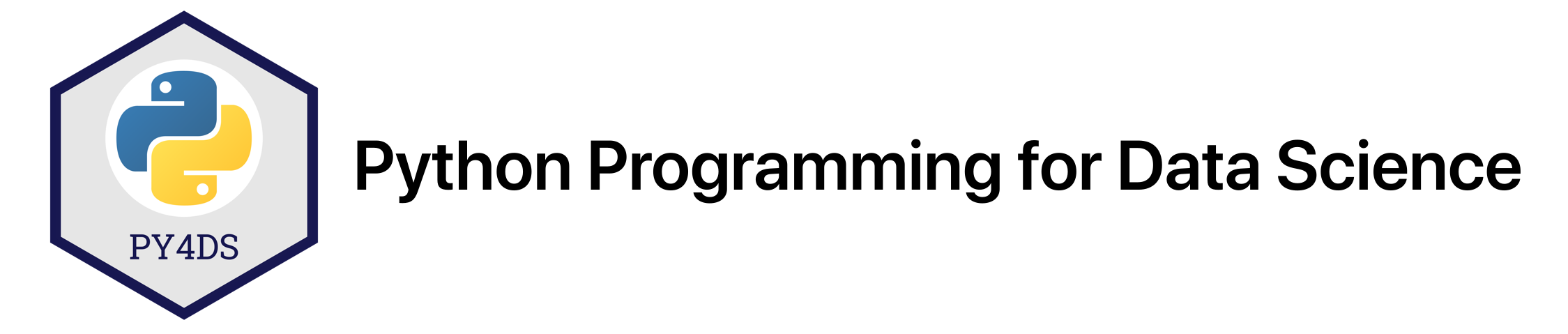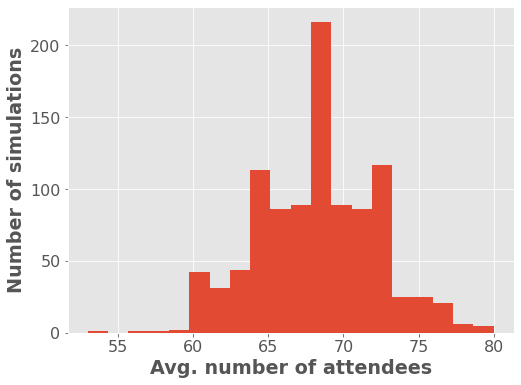# Style Guides, Scripts, Imports¶

Tomas Beuzen, September 2020

These exercises complement Chapter 4.

## Exercises¶

### 1.¶

Use flake8 to list all the syntatic and stylistic problems with the following code. You could copy the code into a .py file and run flake8 from the command line (as was shown in Chapter 4), or you could try the JupyterLab flake8 extension.

very_long_variable_name = {'field': 1,
'is_debug': True}
if very_long_variable_name is not None and very_long_variable_name["field"] > 0 or very_long_variable_name['is_debug']:
z = 'hello '+'world'
else:
f = rf'hello {world}'
if (True): y = 'hello ''world'#FIXME: https://github.com/python/black/issues/26
class Foo  (     object  ):
def f    (self   ):
return       37*-2
def g(self, x,y=42):
return y
regular_formatting = [
0,  1,  2,
3,  4,  5
]
def CAPITALIZE(mystring):
return mystring.upper()

# Your answer here.


### 2.¶

Use black to autoformat the following code. You could copy the code into a .py file and run black from the command line (as was shown in Chapter 4), or you could try the JupyterLab black extension.

very_long_variable_name = {'field': 1,
'is_debug': True}
if very_long_variable_name is not None and very_long_variable_name["field"] > 0 or very_long_variable_name['is_debug']:
z = 'hello '+'world'
else:
f = rf'hello {world}'
if (True): y = 'hello ''world'#FIXME: https://github.com/python/black/issues/26
class Foo  (     object  ):
def f    (self   ):
return       37*-2
def g(self, x,y=42):
return y
regular_formatting = [
0,  1,  2,
3,  4,  5
]
def CAPITALIZE(mystring):
return mystring.upper()

# Your answer here.


### 3.¶

In the last set of practice exercises we created the Circle class:

class Circle:
"""A circle with a radius r."""

def area(self):
"""Calculate the area of the circle."""
return math.pi * self.radius ** 2

def circumference(self):
"""Calculate the circumference of the circle."""
return 2.0 * math.pi * self.radius

def __str__(self):


Save this code into a new Python .py file and then import it here and answer the following:

1. What is the area of a circle with radius 10?

2. What is the circumference of a circle with radius 10?

# Your answer here.


### 4.¶

I recently decided to simulate the possible attendance at a party I was throwing using Python. The idea is that for every guest you invite, you assign them a probability of actually attending your party. For example:

Guest Name

Probability of Attending

Tom

1

0.75

Tiffany

0.5

Joel

0.75

Alexi

0.5

Joel

1

Mike

0.5

Hayley

0.75

In this exercise I want you to create a function that models each guest’s attendance as a Bernoulli random variable. You can run a Bernoulli trial using the numpy library function random.binomial(n=1, ...) (here are the docs). You should import this function and run a single simulation on the list above (note that results may vary as we won’t fix the random seed here).

I’ve given you the list of probabilities to get you started. Your code should output the total number of attendees after running your simulation.

This question is based on a random website post I made recently.

probabilities = [1, 0.75, 0.5, 0.75, 0.5, 1, 0.5, 0.75]



### 5.¶

So after completing Exercise 4, you have a way of running a single simulation given a list of probabilities. Now I want you to write a function called simulate_party(probabilities, n) that runs n simulations for a list of probabilities and returns a list of the total number of attendees for each simulation.

Then, calculate the average guest attendance after running 100 simulations (round up to the nearest integer - hint: math.ceil()).

def simulate_party(probabilities, n):
"Simulate attendance at a party from a list of attendance probabilities."
pass  # Remove this line and add your answer here.


Once you have a working function, uncomment the code below to plot the results of your function on a much longer guest list!

# Use this code to plot your function on a bigger guest list!
import numpy as np
import matplotlib.pyplot as plt
plt.style.use('ggplot')  # These lines are to do with plot formatting. We'll talk about them in a later chapter.
plt.rcParams.update({'font.size': 16, 'axes.labelweight': 'bold', 'figure.figsize': (8, 6)})

number_of_guests=100
probabilities = np.random.choice([0.2, 0.4, 0.6, 0.8, 1],
size=number_of_guests,
p=[0.1, 0.2, 0.2, 0.3, 0.2])

# attendance = simulate_party(probabilities, n=1000)
# plt.hist(attendance, bins=20)
# plt.xlabel("Avg. number of attendees")
# plt.ylabel("Number of simulations");


## Solutions¶

### 1.¶

Use flake8 to list all the syntatic and stylistic problems with the following code. You could copy the code into a .py file and run flake8 from the command line (as was shown in Chapter 4), or you could try the JupyterLab flake8 extension.

very_long_variable_name = {'field': 1,
'is_debug': True}
if very_long_variable_name is not None and very_long_variable_name["field"] > 0 or very_long_variable_name['is_debug']:
z = 'hello '+'world'
else:
f = rf'hello {world}'
if (True): y = 'hello ''world'#FIXME: https://github.com/python/black/issues/26
class Foo  (     object  ):
def f    (self   ):
return       37*-2
def g(self, x,y=42):
return y
regular_formatting = [
0,  1,  2,
3,  4,  5
]
def CAPITALIZE(mystring):
return mystring.upper()

!flake8 bad_style.py

bad_style.py:2:25: E128 continuation line under-indented for visual indent
bad_style.py:3:80: E501 line too long (119 > 79 characters)
bad_style.py:4:2: E111 indentation is not a multiple of four
bad_style.py:6:2: E111 indentation is not a multiple of four
bad_style.py:6:16: F821 undefined name 'world'
bad_style.py:7:10: E701 multiple statements on one line (colon)
bad_style.py:7:31: E261 at least two spaces before inline comment
bad_style.py:8:1: E302 expected 2 blank lines, found 0
bad_style.py:8:13: E201 whitespace after '('
bad_style.py:8:25: E202 whitespace before ')'
bad_style.py:9:3: E111 indentation is not a multiple of four
bad_style.py:9:8: E211 whitespace before '('
bad_style.py:9:19: E202 whitespace before ')'
bad_style.py:10:11: E271 multiple spaces after keyword
bad_style.py:11:3: E301 expected 1 blank line, found 0
bad_style.py:11:3: E111 indentation is not a multiple of four
bad_style.py:11:16: E231 missing whitespace after ','
bad_style.py:12:7: E111 indentation is not a multiple of four
bad_style.py:13:1: E305 expected 2 blank lines after class or function definition, found 0
bad_style.py:17:1: E302 expected 2 blank lines, found 0
bad_style.py:18:28: W292 no newline at end of file


### 2.¶

Use black to autoformat the following code. You could copy the code into a .py file and run black from the command line (as was shown in Chapter 4), or you could try the JupyterLab black extension.

very_long_variable_name = {'field': 1,
'is_debug': True}
if very_long_variable_name is not None and very_long_variable_name["field"] > 0 or very_long_variable_name['is_debug']:
z = 'hello '+'world'
else:
f = rf'hello {world}'
if (True): y = 'hello ''world'#FIXME: https://github.com/python/black/issues/26
class Foo  (     object  ):
def f    (self   ):
return       37*-2
def g(self, x,y=42):
return y
regular_formatting = [
0,  1,  2,
3,  4,  5
]
def CAPITALIZE(mystring):
return mystring.upper()

# I used the Jupyter Lab code formatter extension for this.

very_long_variable_name = {"field": 1, "is_debug": True}
if (
very_long_variable_name is not None
and very_long_variable_name["field"] > 0
or very_long_variable_name["is_debug"]
):
z = "hello " + "world"
else:
f = rf"hello {world}"
if True:
y = "hello " "world"  # FIXME: https://github.com/python/black/issues/26

class Foo(object):
def f(self):
return 37 * -2

def g(self, x, y=42):
return y

regular_formatting = [0, 1, 2, 3, 4, 5]

def CAPITALIZE(mystring):
return mystring.upper()


### 3.¶

In the last set of practice exercises we created the Circle class:

class Circle:
"""A circle with a radius r."""

def area(self):
"""Calculate the area of the circle."""
return math.pi * self.radius ** 2

def circumference(self):
"""Calculate the circumference of the circle."""
return 2.0 * math.pi * self.radius

def __str__(self):


Save this code into a new Python .py file and then import it here and answer the following:

1. What is the area of a circle with radius 10?

2. What is the circumference of a circle with radius 10?

from circle import Circle

c = Circle(10)
print(f"The circle has area = {c.area():.2f}")
print(f"The circle has circumference = {c.circumference():.2f}")

The circle has area = 314.16
The circle has circumference = 62.83


### 4.¶

I recently decided to simulate the possible attendance at a party I was throwing using Python. The idea is that for every guest you invite, you assign them a probability of actually attending your party. For example:

Guest Name

Probability of Attending

Tom

1

0.75

Tiffany

0.5

Joel

0.75

Alexi

0.5

Joel

1

Mike

0.5

Hayley

0.75

In this exercise I want you to create a function that models each guest’s attendance as a Bernoulli random variable. You can run a Bernoulli trial using the numpy library function random.binomial(n=1, ...) (here are the docs). You should import this function and run a single simulation on the list above (note that results may vary as we won’t fix the random seed here).

I’ve given you the list of probabilities to get you started. Your code should output the total number of attendees after running your simulation.

This question is based on a random website post I made recently.

probabilities = [1, 0.75, 0.5, 0.75, 0.5, 1, 0.5, 0.75]

import numpy as np

attendance_simulation = [np.random.binomial(n=1, p=p) for p in probabilities]
print(f"{sum(attendance_simulation)} guests attended the party in this simulation!")

6 guests attended the party in this simulation!


### 5.¶

So after completing Exercise 4, you have a way of running a single simulation given a list of probabilities. Now I want you to write a function that runs n simulations and returns a list of the total number of attendees for each simulation. Calculate the average guest attendance after running 100 simulations (round up to the nearest integer - hint: math.ceil()).

import math

def simulate_party(probabilities, n):
"Simulate attendance at a party from a list of attendance probabilities."

attendance = []
for i in range(n):
attendance.append(sum([np.random.binomial(n=1, p=p) for p in probabilities]))
return attendance

simulations = simulate_party(probabilities, 100)

print(
f"Avg. number of guests across all simulations: {math.ceil(sum(simulations) / len(simulations))}"
)

Avg. number of guests across all simulations: 6

# Use this code to plot your function on a bigger guest list!
import numpy as np
import matplotlib.pyplot as plt
plt.style.use('ggplot')  # These lines are to do with plot formatting. We'll talk about them in a later chapter.
plt.rcParams.update({'font.size': 16, 'axes.labelweight': 'bold', 'figure.figsize': (8, 6)})

number_of_guests=100
probabilities = np.random.choice([0.2, 0.4, 0.6, 0.8, 1],
size=number_of_guests,
p=[0.1, 0.2, 0.2, 0.3, 0.2])
attendance = simulate_party(probabilities, n=1000)
plt.hist(attendance, bins=20)
plt.xlabel("Avg. number of attendees")
plt.ylabel("Number of simulations");